## 2.3.1 The magnetization dynamics and the Landau-Lifshitz-Gilbert equation

The equation of motion of a magnetic moment under an applied field is(2.39)

whereis the gyromagnetic ratio for a Landé factor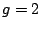. The moment precesses around the field with the Larmor frequency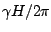and never reaches the equilibrium position unless it already points in the same direction. The equation conserves both the energy and the magnetic moment magnitude. The macroscopic magnetization vector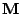also satisfies a similar equation, recalling Eq. (2.13). The equation is valid from both the micromagnetic and the atomistic point of view and the way to include any interaction in the equation is to use instead of the external field the effective field calculated by means of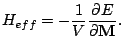(2.40)

Eq. (2.39) does not explain the absorption line in resonance experiments and the fact that the magnetic moment eventually aligns with field direction. The motion is modified by the interaction with crystal lattice vibrations, conduction electrons and other external sources. L.D Landau and E.M. Lifshitz [Landau 35] proposed to add a term proportional to, which conserves the magnitude, in order to obtain a phenomenological equation of the spin dynamics. Posteriorly, T. Gilbert suggested to add a viscous forceto the equation of motion (2.39) [Gilbert 55]. The Gilbert and the Landau-Lifshitz equations are equivalent with the renormalization of the precession and dissipation terms. The Gilbert version is preferred because it predicts slower motion with increasing damping. The Gilbert equation, converted to Landau-Lifshitz form, is known as the Landau-Lifshitz-Gilbert(LLG) equation and has the expression: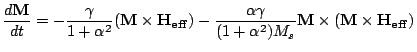(2.41)

where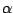is the phenomenological damping constant, which represents all the relaxation mechanisms. For longitudinal magnetic recording media the damping parameter measured by ferromagnetic resonance lays in the range of[Inaba 97]. The equation is dissipative and does not conserve the energy, albeit conserving the magnitude of the magnetization. An example of dissipative LLG dynamics can be seen in Fig. 2.9. The precession frequency in the absence of external field is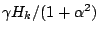, therefore, sometimes it is useful to express the equation in reduced units(2.42)

where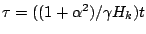is the reduced time,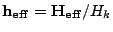is the reduced field andthe reduced magnetization.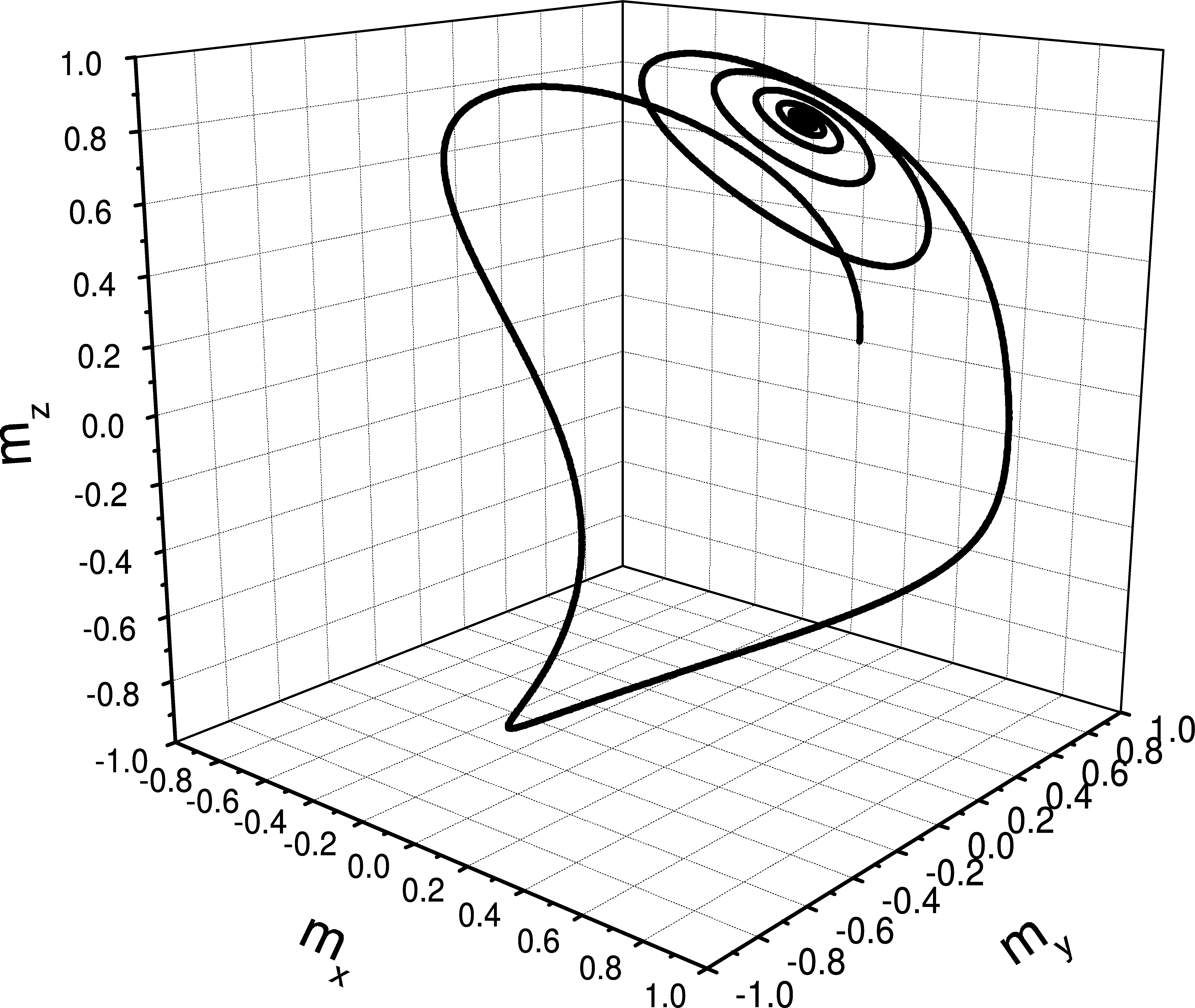The second term of right side of Eq. (2.41), the damping term, makes the magnetization rotate towards the direction of the effective field and eventually to be parallel to its direction, reaching the equilibrium. That represents a minimum of the energy. Accordingly, we can use the integration of LLG equation to minimize the energy [Berkov 93] and this is the method we mostly use in this thesis. For this purpose, it is better to use a large value of the damping constant and to remove the precession term.

Subsections
2008-04-04